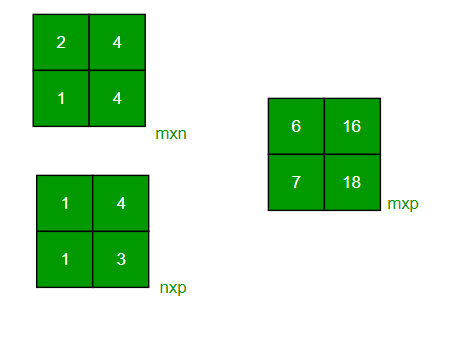GeeksforGeeks App
Open AppBrowser
Continue

# Python Program to multiply two matrices

Given two matrices, the task to multiply them. Matrices can either be square or rectangular.

Examples:

```Input : mat1[][] = {{1, 2},
{3, 4}}
mat2[][] = {{1, 1},
{1, 1}}
Output : {{3, 3},
{7, 7}}
Input : mat1[][] = {{2, 4},
{3, 4}}
mat2[][] = {{1, 2},
{1, 3}}
Output : {{6, 16},
{7, 18}}```Multiplication of Square Matrices :
The below program multiplies two square matrices of size 4*4, we can change N for different dimensions.

## Python3

 `# 4x4 matrix multiplication using Python3``# Function definition``def` `matrix_multiplication(M, N):``    ``# List to store matrix multiplication result``    ``R ``=` `[[``0``, ``0``, ``0``, ``0``],``        ``[``0``, ``0``, ``0``, ``0``],``        ``[``0``, ``0``, ``0``, ``0``],``        ``[``0``, ``0``, ``0``, ``0``]]` `    ``for` `i ``in` `range``(``0``, ``4``):``        ``for` `j ``in` `range``(``0``, ``4``):``            ``for` `k ``in` `range``(``0``, ``4``):``                ``R[i][j] ``+``=` `M[i][k] ``*` `N[k][j]` `    ``for` `i ``in` `range``(``0``, ``4``):``        ``for` `j ``in` `range``(``0``, ``4``):``            ``# if we use print(), by default cursor moves to next line each time,``            ``# Now we can explicitly define ending character or sequence passing``            ``# second parameter as end =""``            ``# syntax: print(, end ="")``            ``# Here space (" ") is used to print a gap after printing``            ``# each element of R``            ``print``(R[i][j], end ``=``" "``)``        ``print``("``", end ="``")` `# First matrix. M is a list``M ``=` `[[``1``, ``1``, ``1``, ``1``],``    ``[``2``, ``2``, ``2``, ``2``],``    ``[``3``, ``3``, ``3``, ``3``],``    ``[``4``, ``4``, ``4``, ``4``]]` `# Second matrix. N is a list``N ``=` `[[``1``, ``1``, ``1``, ``1``],``    ``[``2``, ``2``, ``2``, ``2``],``    ``[``3``, ``3``, ``3``, ``3``],``    ``[``4``, ``4``, ``4``, ``4``]]``    ` `# Call matrix_multiplication function``matrix_multiplication(M, N)` `# This code is contributed by Santanu`

Output

```Result matrix is
10 10 10 10
20 20 20 20
30 30 30 30
40 40 40 40```

Time complexity: O(n3). It can be optimized using Strassen’s Matrix Multiplication

Auxiliary Space: O(n2)

Multiplication of Rectangular Matrices :
We use pointers in C to multiply to matrices. Please refer to the following post as a prerequisite of the code.
How to pass a 2D array as a parameter in C?

## Python3

 `# Python3 program to multiply two``# rectangular matrices` `# Multiplies two matrices mat1[][]``# and mat2[][] and prints result.``# (m1) x (m2) and (n1) x (n2) are``# dimensions of given matrices.``def` `multiply(m1, m2, mat1,``             ``n1, n2, mat2):` `    ``res ``=` `[[``0` `for` `x ``in` `range``(n2)]``              ``for` `y ``in` `range` `(m1)]``    ` `    ``for` `i ``in` `range``(m1):``        ``for` `j ``in` `range``(n2):``            ``res[i][j] ``=` `0``            ``for` `x ``in` `range``(m2):           ``                ``res[i][j] ``+``=` `(mat1[ i][x] ``*``                              ``mat2[ x][j])``           ` `    ``for` `i ``in` `range``(m1):``        ``for` `j ``in` `range``(n2):     ``            ``print` `(res[i][j],``                   ``end ``=` `" "``)       ``        ``print` `()` `# Driver code``if` `__name__ ``=``=` `"__main__"``:``  ` `    ``mat1 ``=` `[[``2``, ``4``], [``3``, ``4``]]``    ``mat2 ``=` `[[``1``, ``2``], [``1``, ``3``]]``    ``m1, m2, n1, n2 ``=` `2``, ``2``, ``2``, ``2``  ` `    ``# Function call``    ``multiply(m1, m2, mat1,``             ``n1, n2, mat2)``    ` `# This code is contributed by Chitranayal`

Output

```6 16
7 18```

Time complexity: O(n3). It can be optimized using Strassen’s Matrix Multiplication

Auxiliary Space: O(m1 * n2)

Please refer complete article on Program to multiply two matrices for more details!

My Personal Notes arrow_drop_up